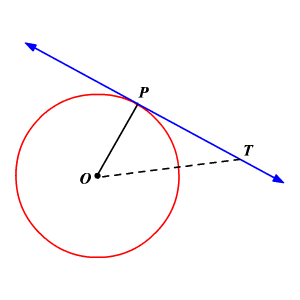# Tangent to a Circle

A tangent to a circle is a straight line which touches the circle at only one point. This point is called the point of tangency.

The tangent to a circle is perpendicular to the radius at the point of tangency.In the circle $O$ , $\stackrel{↔}{PT}$ is a tangent and $\stackrel{¯}{OP}$ is the radius.

If $\stackrel{↔}{PT}$ is a tangent, then $\stackrel{¯}{OP}$ is perpendicular to $\stackrel{↔}{PT}$ .

For example, suppose $\stackrel{¯}{OP}$ $=3$ units and $\stackrel{¯}{PT}$ $=4$ units. Find the length of $\stackrel{¯}{OT}$ .

Because the radius is perpendicular to the tangent at the point of tangency, $\stackrel{¯}{OP}\perp \stackrel{↔}{PT}$ .

This makes the angle $P$ a right angle in the triangle $OPT$ and triangle $OPT$ a right triangle.

Now use the Pythagorean Theorem to find $\stackrel{¯}{OT}$ .

$\begin{array}{l}{\left(OP\right)}^{2}+{\left(PT\right)}^{2}={\left(OT\right)}^{2}\\ \text{\hspace{0.17em}}\text{\hspace{0.17em}}\text{\hspace{0.17em}}\text{\hspace{0.17em}}\text{\hspace{0.17em}}\text{\hspace{0.17em}}\text{\hspace{0.17em}}\text{\hspace{0.17em}}\text{\hspace{0.17em}}\text{\hspace{0.17em}}\text{\hspace{0.17em}}\text{\hspace{0.17em}}\text{\hspace{0.17em}}\text{\hspace{0.17em}}\text{\hspace{0.17em}}\text{\hspace{0.17em}}{3}^{2}+{4}^{2}={\left(OT\right)}^{2}\\ \text{\hspace{0.17em}}\text{\hspace{0.17em}}\text{\hspace{0.17em}}\text{\hspace{0.17em}}\text{\hspace{0.17em}}\text{\hspace{0.17em}}\text{\hspace{0.17em}}\text{\hspace{0.17em}}\text{\hspace{0.17em}}\text{\hspace{0.17em}}\text{\hspace{0.17em}}\text{\hspace{0.17em}}\text{\hspace{0.17em}}\text{\hspace{0.17em}}\text{\hspace{0.17em}}\text{\hspace{0.17em}}\text{\hspace{0.17em}}\text{\hspace{0.17em}}9+16={\left(OT\right)}^{2}\\ \text{\hspace{0.17em}}\text{\hspace{0.17em}}\text{\hspace{0.17em}}\text{\hspace{0.17em}}\text{\hspace{0.17em}}\text{\hspace{0.17em}}\text{\hspace{0.17em}}\text{\hspace{0.17em}}\text{\hspace{0.17em}}\text{\hspace{0.17em}}\text{\hspace{0.17em}}\text{\hspace{0.17em}}\text{\hspace{0.17em}}\text{\hspace{0.17em}}\text{\hspace{0.17em}}\text{\hspace{0.17em}}\text{\hspace{0.17em}}\text{\hspace{0.17em}}\text{\hspace{0.17em}}\text{\hspace{0.17em}}\text{\hspace{0.17em}}\text{\hspace{0.17em}}\text{\hspace{0.17em}}\text{\hspace{0.17em}}25={\left(OT\right)}^{2}\\ \text{\hspace{0.17em}}\text{\hspace{0.17em}}\text{\hspace{0.17em}}\text{\hspace{0.17em}}\text{\hspace{0.17em}}\text{\hspace{0.17em}}\text{\hspace{0.17em}}\text{\hspace{0.17em}}\text{\hspace{0.17em}}\text{\hspace{0.17em}}\text{\hspace{0.17em}}\text{\hspace{0.17em}}\text{\hspace{0.17em}}\text{\hspace{0.17em}}\text{\hspace{0.17em}}\text{\hspace{0.17em}}\text{\hspace{0.17em}}\text{\hspace{0.17em}}\text{\hspace{0.17em}}\text{\hspace{0.17em}}\text{\hspace{0.17em}}\text{\hspace{0.17em}}±5=OT\end{array}$

Since the length cannot be negative, the length of $\stackrel{¯}{OT}$ is $5$ units.